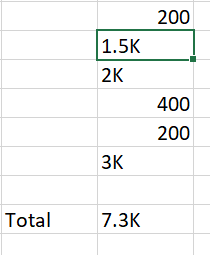# I want to do sum of variables which is in form of 1k,2k,400,200 etc

Hello Team,

I am pulling some values from get text and need to do sum but the values format is different. Some values in normal values and some values comes K.
Eg- 500,1k,200,300,2k
Total of above numbers is->4K.

I want total in K format not in all numbers.@Jeeru_venkat_Rao

Quick question when you read the data can you see how youa re getting it…this can be seen from locals panel after reading data into datatable…try using raw values option in use excel file

I believe this is the excel cell format and when you read you would get the data normally

cheers

@Anil_G , I just use excel to show you how the values comes and how I need output. Basically I pull all those values through Get Text activity and make the sum like in Excel.

@Jeeru_venkat_Rao

`sumvalue = (dt.AsEnumerable.Sum(function(x) CDBL(If(IsNumeric(x("ColumnName").ToString),x("ColumnName").ToString,(CDBL(x("ColumnName").ToString.Replace("K",""))*1000).ToString))/1000).ToString + "K"`

sumvalue will be in string format

cheers

Hi,
You can check for the existence of K in the value. If K is present, just replace it with nothing and convert the rest of the value to number format and multiply it by 1000. If K is not present you can simply add it to other numbers but make sure to convert it to number format if it is string.

So you can, check the value that you’re retrieving from get text activity, suppose you’re getting that in strData.
I’m creating a Amount Value of Double type.
also creating a variable called sum of double type
sum=0
if strData.toLower.contains(“k”)
then
Amount= CDbl(strData.toLower.replace(“k”,“”))*1000
sum=sum+amount
else
sum=sum+amount.

That way you can insert a numeric value in the excel.
Also the sum could be performed in the if condition itself.

Thanks

Thanks to all of you for your quick responses.

This topic was automatically closed 3 days after the last reply. New replies are no longer allowed.